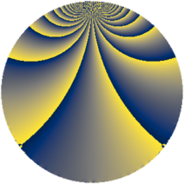# Properties

 Label 138.2.fLevel $138$ Weight $2$ Character orbit 138.f Rep. character $\chi_{138}(5,\cdot)$ Character field $\Q(\zeta_{22})$ Dimension $80$ Newform subspaces $1$ Sturm bound $48$ Trace bound $0$

# Related objects

## Defining parameters

 Level: $$N$$ $$=$$ $$138 = 2 \cdot 3 \cdot 23$$ Weight: $$k$$ $$=$$ $$2$$ Character orbit: $$[\chi]$$ $$=$$ 138.f (of order $$22$$ and degree $$10$$) Character conductor: $$\operatorname{cond}(\chi)$$ $$=$$ $$69$$ Character field: $$\Q(\zeta_{22})$$ Newform subspaces: $$1$$ Sturm bound: $$48$$ Trace bound: $$0$$

## Dimensions

The following table gives the dimensions of various subspaces of $$M_{2}(138, [\chi])$$.

Total New Old
Modular forms 280 80 200
Cusp forms 200 80 120
Eisenstein series 80 0 80

## Trace form

 $$80 q + 4 q^{3} + 8 q^{4} + 4 q^{6} + O(q^{10})$$ $$80 q + 4 q^{3} + 8 q^{4} + 4 q^{6} - 4 q^{12} + 8 q^{13} - 22 q^{15} - 8 q^{16} - 28 q^{18} - 66 q^{21} - 4 q^{24} - 48 q^{25} - 38 q^{27} - 44 q^{30} - 16 q^{31} - 22 q^{33} - 44 q^{37} - 24 q^{39} - 44 q^{43} - 16 q^{46} + 4 q^{48} - 76 q^{49} - 8 q^{52} - 6 q^{54} + 64 q^{55} + 66 q^{57} + 36 q^{58} + 22 q^{60} + 88 q^{61} + 110 q^{63} + 8 q^{64} + 88 q^{66} + 44 q^{67} + 82 q^{69} + 112 q^{70} + 28 q^{72} + 52 q^{73} + 136 q^{75} + 82 q^{78} + 88 q^{79} + 36 q^{81} + 44 q^{82} + 22 q^{84} + 20 q^{85} - 10 q^{87} + 8 q^{93} - 56 q^{94} + 4 q^{96} - 132 q^{97} - 66 q^{99} + O(q^{100})$$

## Decomposition of $$S_{2}^{\mathrm{new}}(138, [\chi])$$ into newform subspaces

Label Dim $A$ Field CM Traces $q$-expansion
$a_{2}$ $a_{3}$ $a_{5}$ $a_{7}$
138.2.f.a $80$ $1.102$ None $$0$$ $$4$$ $$0$$ $$0$$

## Decomposition of $$S_{2}^{\mathrm{old}}(138, [\chi])$$ into lower level spaces

$$S_{2}^{\mathrm{old}}(138, [\chi]) \cong$$ $$S_{2}^{\mathrm{new}}(69, [\chi])$$$$^{\oplus 2}$$